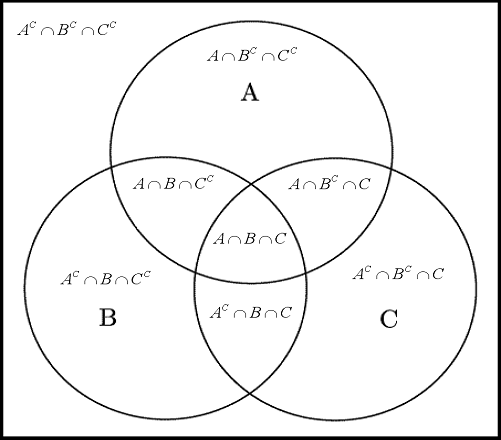# Venn Diagram And Operation On Sets

Approved & Edited by ProProfs Editorial Team
The editorial team at ProProfs Quizzes consists of a select group of subject experts, trivia writers, and quiz masters who have authored over 10,000 quizzes taken by more than 100 million users. This team includes our in-house seasoned quiz moderators and subject matter experts. Our editorial experts, spread across the world, are rigorously trained using our comprehensive guidelines to ensure that you receive the highest quality quizzes.
| By Jaksiboy
J
Jaksiboy
Community Contributor
Quizzes Created: 919 | Total Attempts: 12,132,600
Questions: 10 | Attempts: 3,168SettingsAre you a math geek or consider yourself to be one? What do you know about this aspect in mathematics? Take this quiz to find out.

• 1.

### A venn diagram is also called a

• A.

Graph

• B.

Circle

• C.

Set diagram

• D.

Figure

C. Set diagram
Explanation
A Venn diagram is a graphical representation used to show the relationships between different sets of items. It consists of overlapping circles or ellipses, where each circle represents a set and the overlapping area represents the intersection of those sets. Therefore, the term "set diagram" is an appropriate alternative name for a Venn diagram.

Rate this question:

• 2.

### A venn diagram uses circles that

• A.

Overlap

• B.

Broken

• C.

Spaced

• D.

Figured

A. Overlap
Explanation
A Venn diagram uses circles that overlap to represent the relationship between different sets or groups. The overlapping area indicates the elements that are common to both sets, while the non-overlapping areas represent elements that are unique to each set. This visual representation helps to illustrate the similarities and differences between different groups or categories.

Rate this question:

• 3.

### Items inside a venn diagram are called

• A.

Images

• B.

Figures

• C.

Elements

• D.

Numbers

C. Elements
Explanation
The correct answer is "elements" because in a Venn diagram, the circles or shapes represent sets, and the items or objects within those shapes are referred to as elements. Each element belongs to one or more sets, and the Venn diagram visually represents the relationships between these sets and their elements.

Rate this question:

• 4.

### Who invented the diagram?

• A.

John Venn

• B.

Einstein

• C.

Newton

• D.

Carlos John

A. John Venn
Explanation
John Venn is credited with inventing the diagram. The Venn diagram is a visual representation used to illustrate the relationships between different sets or groups of objects. It consists of overlapping circles or other shapes that represent the different sets, with the overlapping areas indicating the elements that belong to multiple sets. John Venn, a British mathematician and logician, introduced this method of diagrammatic representation in the late 19th century. The Venn diagram has since become a widely used tool in various fields, including mathematics, logic, statistics, and computer science.

Rate this question:

• 5.

### What year was the diagram invented?

• A.

1990

• B.

1880

• C.

1889

• D.

1993

B. 1880
Explanation
The correct answer is 1880. This suggests that the diagram was invented in the year 1880.

Rate this question:

• 6.

### The sum of all sets is called

• A.

Extra

• B.

All

• C.

Union

• D.

C. Union
Explanation
The term "union" refers to the sum of all sets. It is a mathematical operation that combines all the elements from multiple sets into a single set, without any repetition. In this context, "extra," "all," and "add" do not accurately describe the sum of all sets. Therefore, the correct answer is "union."

Rate this question:

• 7.

### Elements common to two sets are called

• A.

Intersection

• B.

Member

• C.

Union

• D.

Venn

A. Intersection
Explanation
The term "intersection" refers to the elements that are common to both sets. It is the shared portion or overlap between the two sets. In this case, the correct answer is "intersection" because it accurately describes the concept of elements that are present in both sets.

Rate this question:

• 8.

### Points outside the diagram are called

• A.

Invalid

• B.

Removal

• C.

Non-set

• D.

Never

C. Non-set
Explanation
Points outside the diagram are referred to as "non-set" because they do not belong to the set or region represented by the diagram. These points are not included or considered within the boundaries or constraints of the diagram, and therefore are classified as non-set points.

Rate this question:

• 9.

### Venn diagram is used to teach

• A.

Math

• B.

Set theory

• C.

Art

• D.

History

B. Set theory
Explanation
A Venn diagram is a visual representation used to illustrate relationships between different sets or groups. It consists of overlapping circles or shapes that represent the sets, with the overlapping regions indicating the elements that belong to multiple sets. Set theory is a branch of mathematics that deals with the study of sets, their properties, and the relationships between them. Therefore, a Venn diagram is commonly used as a teaching tool in mathematics to help students understand and visualize concepts related to set theory.

Rate this question:

• 10.

### Sets are represented by

• A.

Figures

• B.

Letters

• C.

Graphs

• D.

Drawing

B. Letters
Explanation
Sets are typically represented by letters. In mathematics, sets are a collection of distinct objects, and each object is represented by a letter. For example, if we have a set of numbers {1, 2, 3}, we can represent it as A = {1, 2, 3}, where A is the name of the set. Using letters to represent sets allows for easy identification and manipulation of the objects within the set. Figures, graphs, and drawings may be used to visually represent sets in certain contexts, but letters are the most common and standard way to represent sets.

Rate this question:

Related TopicsBack to top